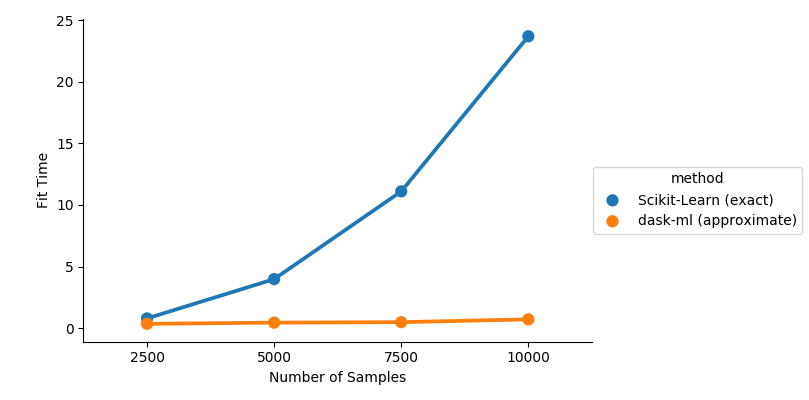# Spectral Clustering Example¶

This example shows how dask-ml’s `SpectralClustering` scales with the number of samples, compared to scikit-learn’s implementation. The dask version uses an approximation to the affinity matrix, which avoids an expensive computation at the cost of some approximation error.```from sklearn.datasets import make_circles
from sklearn.utils import shuffle
import pandas as pd

from timeit import default_timer as tic
import sklearn.cluster
import seaborn as sns

Ns = [2500, 5000, 7500, 10000]
X, y = make_circles(n_samples=10_000, noise=0.05, random_state=0, factor=0.5)
X, y = shuffle(X, y)

timings = []
for n in Ns:
X, y = make_circles(n_samples=n, random_state=n, noise=0.5, factor=0.5)
t1 = tic()
sklearn.cluster.SpectralClustering(n_clusters=2).fit(X)
timings.append(('Scikit-Learn (exact)', n, tic() - t1))
t1 = tic()